# What Is the Necessity of Bias in Neural Networks?The concept of neural networks or artificial neural networks (ANNs) in the field of artificial intelligence originated from the manner in which biological systems process information. Unlike biological systems, however, they are efficient models for statistical pattern recognition as they don’t impose unnecessary constraints. ANNs have several components among which weight and bias are the key components. In this article, we will explore the importance of several parameters, with particular emphasis on weights and neural network bias.

## Components in artificial neural networks

ANNs comprise several components like the ones below.

1. Inputs: They’re usually represented as features of a dataset which are passed on to a neural network to make predictions.
2. Weights: These are the real values associated with the features. They are significant as they tell the importance of each feature which is passed as an input to the artificial neural network.
3. Bias: Bias in a neural network is required to shift the activation function across the plane either towards the left or the right. We will cover it in more detail later.
4. Summation function: It is defined as the function which sums up the product of the weight and the features with bias.
5. Activation function: It is required to add non-linearity to the neural network model.

## Creation of a neural network

To construct a neural network, consider M linear combinations of the input variables x1,...,xD in the form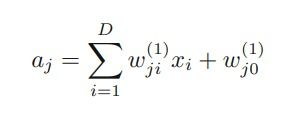where j=1,…,M and the superscript (1) represent the first layer of the neural network. It indicates that the parameters are in the first layer of the network. Further, wji and wj0 represent weights and biases, respectively. The parameters aj are known as activations. Each of these activations are transformed using a differentiable, nonlinear activation function h(.) as shown below: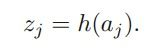The above parameter zj is known as hidden units. The non-linear function h(.) can be a logistic sigmoidal function or ‘tanh’ function. These values are again linearly combined to give output unit activations as below: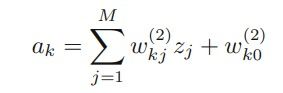After combining the above equations, the overall equation can be written in the form as shown below: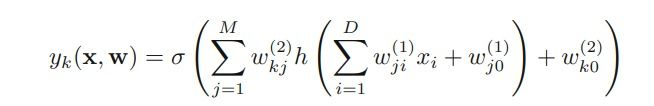## What is bias in a neural network?

In simple words, neural network bias can be defined as the constant which is added to the product of features and weights. It is used to offset the result. It helps the models to shift the activation function towards the positive or negative side.

Let us understand the importance of bias with the help of an example.

Consider a sigmoid activation function which is represented by the equation below: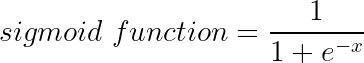On replacing the variable ‘x’ with the equation of line, we get the following: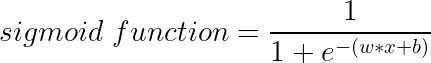In the above equation, ‘w’ is weights, ‘x’ is the feature vector, and ‘b’ is defined as the bias. On substituting the value of ‘b’ equal to 0, we get the graph of the above equation as shown in the figure below: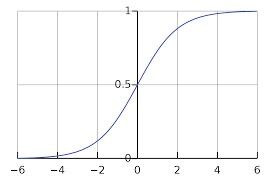Image source: Wikimedia

If we vary the values of the weight ‘w’, keeping bias ‘b’=0, we will get the following graph: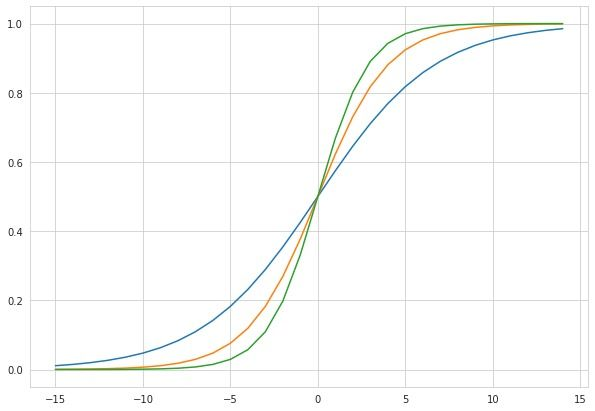Image source: Medium

While changing the values of ‘w’, there is no way we can shift the origin of the activation function, i.e., the sigmoid function. On changing the values of ‘w’, only the steepness of the curve will change. There is only one way to shift the origin and that is to include bias ‘b’.

On keeping the value of weight ‘w’ fixed and varying the value of bias ‘b’, we will get the graph below: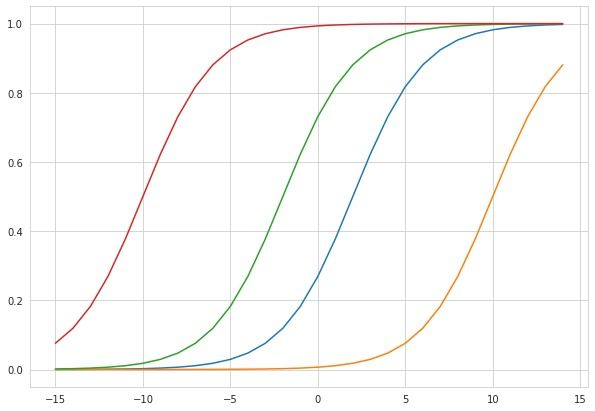Image source: Medium

From the graph, it can be concluded that the bias is required for shifting the origin of the curve to the left or right.

### How to shift the curve to the left or the right

If we substitute the value of ‘w’= -1 and ‘b’=0 in Eq.(6), the graph of the activation function will look as shown below.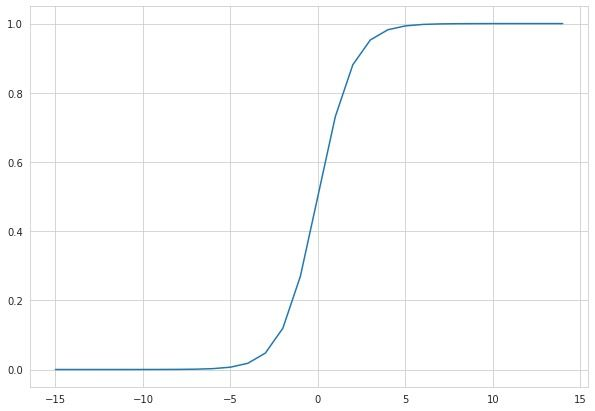Image source: Medium

It can be seen that the output is equal to 0 for the values of ‘x’ less than 0 and equal to 1 for the values of ‘x’ greater than 0.

Now, how should we design our equation so that the output of the activation function is equal to 1 for all values of ‘x’ less than 5? We achieve this by introducing the term bias ‘b’ in our equation with value of ‘b’=-5.

## Why is bias added in neural networks?

To understand the concept of neural network bias, let’s begin by discussing single layer neural networks.

A given neural network computes the function Y=f(X), where X and Y are feature vector and output vector, respectively, with independent components. If the given neural network has weight ‘W’ then it can also be represented as Y=f(X,W). If the dimensionality of both X and Y is equal to 1, the function can be plotted in a two-dimensional plane as below: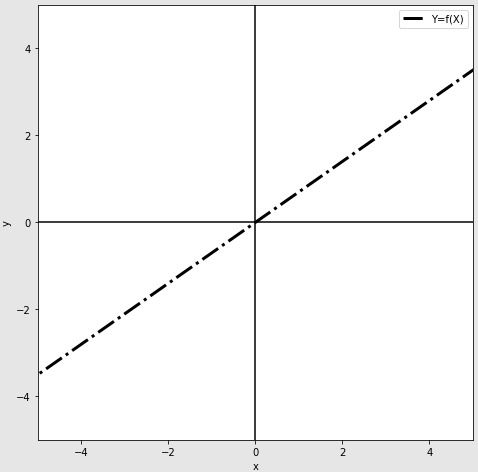Image source: Baeldung

Such a neural network can approximate any linear function of the form y=mx + c. When c=0, then y=f(x)=mx and the neural network can approximate only the functions passing through origin. If a function includes the constant term c, it can approximate any of the linear functions in the plane.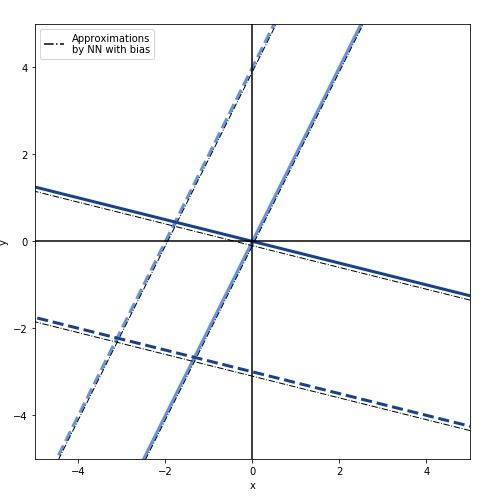Image source: Baeldung

## How to add bias to neural networks

Consider the conditions mentioned in the previous section for the neural network. From there, we can infer that if there is any error during the prediction by the function, bias ‘b’ can be added to the output values for obtaining the true values. Thus, the neural network would compute the function y=f(x) + b which includes all the predictions by the neural network shifted by the constant ‘b’.

Now, if we add one more input to the neural network layer, the function is defined as y=f(x1,x2). Since both x1 and x2 are independent components, they should have independent biases b1 and b2, respectively. Thus, the neural networks can be represented as y=f(x1, x2) + b1 + b2.

### One bias per layer in a neural network

In this section, we’ll discuss whether the bias in a neural network is unique or not. Consider a feature vector X =[x1, x2, ….,xn] and their associated systematic errors (b1+ b2+ ….bn) for n inputs. Thus, a single layer neural network computing a function Y =f(X,W) + (b1+ b2+ ….bn), where W is a weight matrix.

It is known that the linear combination of scalers is a scalar, therefore the linear combination of (b1+ b2+ ….bn) can be combined into a single value as ‘b’. Thus, the equation can be written as Y =f(X,W) + b. This argument demonstrates that there is only bias which is unique to every layer of the neural network.

Now, let’s consider a neural network with a number of layers greater than 1 in the next section.

### One vector of biases in a neural network

Consider a neural network of k layers. Let’s recall the final equation of the function computed by the neural network:We can consider a function: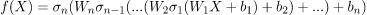Here, we call B=[b1, b2, ….bn], which is a vector consisting of biases of all layers in a neural network. B is considered to be unique as it contains unique values.

Considering f(0) not equal to 0 and the activation functions are odd, as in the case of tanh, the neural network must include bias vector B or it will diverge from the true values. Until the activation functions included in the neural network are odd functions and the function which is being calculated is in a subspace of the feature vector, it becomes mandatory to add non-zero bias vectors in the architecture of the neural network.

### Including bias within the activation function

What happens if we include the bias term within the activation function itself instead of in the output? If the activation function used in the neural network is linear, the neurons will be inactive frequently when their input assumes some values. For instance, consider an activation function as ReLU function which is represented as follows:

ReLU(x) = y = max(0, x)

The graph of the ReLU function is shown below: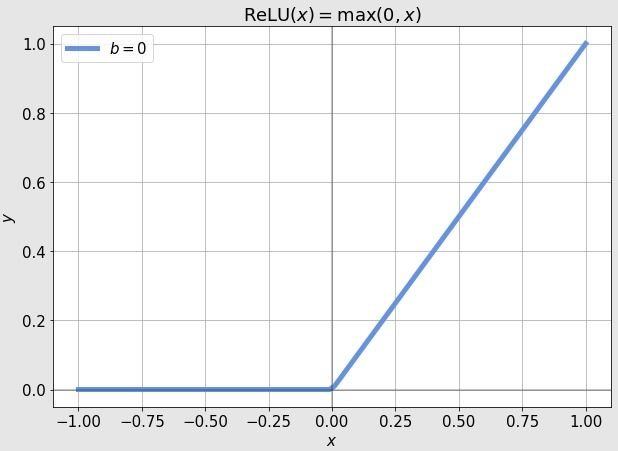Image source: Baeldung

For all the values of x less than or equal to 0, the neural network will have the issue of vanishing gradient. This occurs when the input approaches zero or negative and the gradient approaches zero. Thus, the network cannot perform backpropagation and cannot learn. This problem is not restricted to ReLU but also applies to other activation functions like sigmoid, tanh, etc.

If we include the bias term ‘b’ in the activation function, it would allow the neural network to shift the activation function to the left and to the right by simply modifying the values of b. It would allow the initialization of the derivatives of the error function to non-zero for the values of x between 1 and 0, as shown below: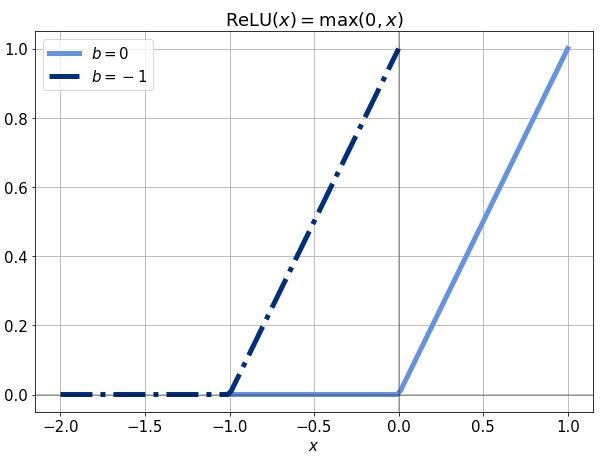Image source: Baeldung

This will allow neurons to be activated even when the input is zero. This in turn will allow backpropagation to occur even when input is zero.

## Types of bias in machine learning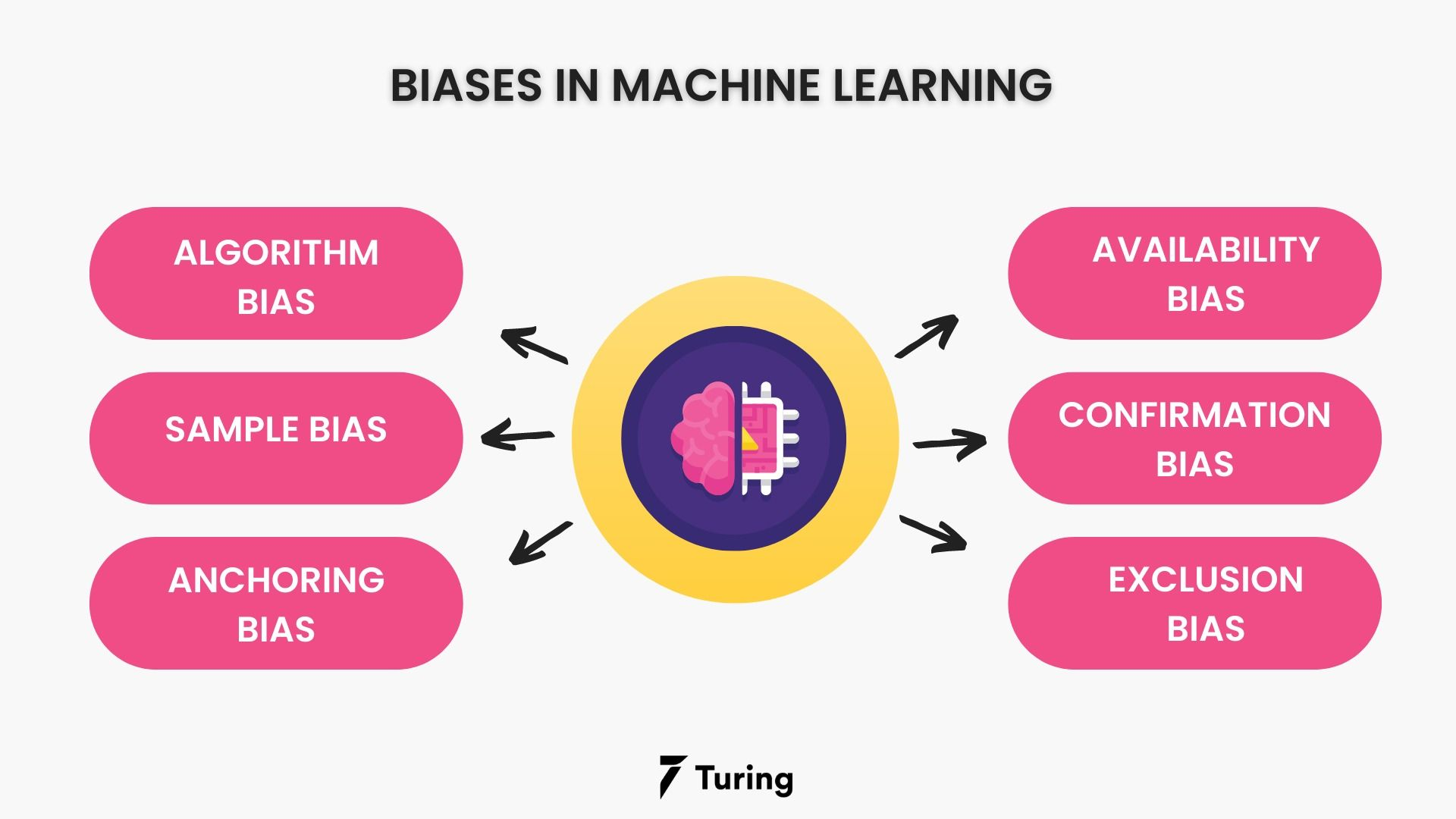The following are the types of biases involved in machine learning:

### Algorithm bias

This kind of bias is introduced into the machine learning pipeline when the algorithms performing the computation are not well written and thus, don’t perform well on the dataset.

### Sample bias

This involves a problem with the training dataset: an imbalance towards a particular class in case of classification problems or insufficient data points for the model to train its algorithm.

### Anchoring bias

This occurs when the data and the choice of metrics are based on personal experience or choice. The models trained on these types of datasets lead to invalid results owing to the preferred standard of the data. They are also hard to discover.

### Availability bias

This kind of bias occurs when the dataset is prepared by the modeler, including the data that the modeler is aware of. For example, in the field of healthcare, if a dataset is trained on a particular comorbidity, it can’t be used to make predictions for something else.

### Confirmation bias

This occurs when the modeler tends to choose the data so that the results are aligned with currently held beliefs, hence leading to bad outcomes when making predictions.

### Exclusion bias

It occurs when the modeler leaves out important data points during the training of the model.

## Bias in deep learning: A case study

A 2019 research paper on ‘Potential Biases in Machine Learning Algorithms Using Electronic Health Record Data’ discusses how bias can impact deep learning algorithms used in the field of healthcare. The paper explores three categories of bias which are mentioned below:

1. Missing Data and Patients Not Identified by Algorithms: They occur due to known or unknown gaps in datasets used to train machine learning algorithms. These gaps could be due to inconsistent data format from the source of information, for example, electronic health records.

2. Sample Size and Underestimation: They occur due to lack of patient sample size, ultimately leading to biased data. For instance, not including patients from a particular race or ethnic group could be a major source of introducing bias in the dataset.

3. Misclassification and Measurement Errors: Quality of data or inconsistency in data being entered by health workers can be a source of introducing bias in the training dataset.

## Ways to reduce bias in deep learning

The following methods can reduce bias in deep learning:

1. Choosing the correct machine learning model.
2. The training dataset has no class imbalance.
3. Data processing is performed mindfully.
4. There is no data leakage in the machine learning pipeline.

In this article, we discussed the formulation of neural networks, the architecture of neural networks, the role of activation functions, and bias - the constant terms that are introduced to reduce errors in neural networks. They can also be introduced in activation functions. We also studied their impact on neural networks and how they can lead to inaccuracy in model training if not taken seriously. As discussed, there are several ways to prevent this inaccuracy, such as choosing the right ML model after carefully analyzing the data or performing correct operations during data preprocessing.

## Author

•### Turing

Author is a seasoned writer with a reputation for crafting highly engaging, well-researched, and useful content that is widely read by many of today's skilled programmers and developers.

Bias allows for the reduction of error during computation by activation functions. They also result in non null output in case of null input.

Weights are vital as they tell the importance of a feature vector and the relationship between a particular feature in the dataset and the target value.

The value of bias in deep learning is usually taken as 1.### Press

What's up with Turing? Get the latest news about us here.### Blog